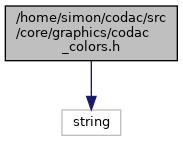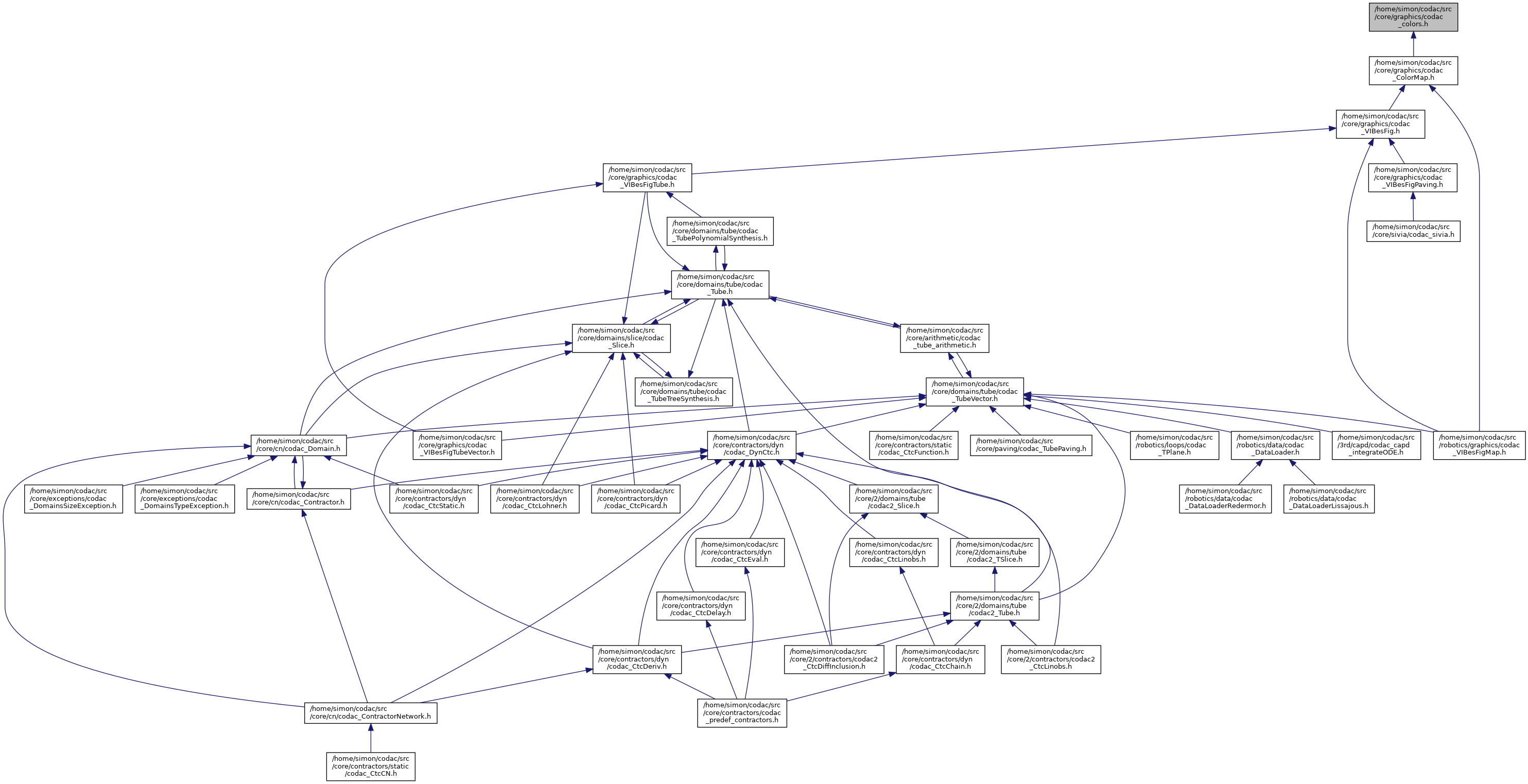codac
codac_colors.h File Reference
#include <string>
Include dependency graph for codac_colors.h:This graph shows which files directly or indirectly include this file:Go to the source code of this file.

## Classes

struct  codac::rgb
Represents an RGB value. More...

struct  codac::hsv
Represents an HSV value. More...

## Namespaces

codac
FixPoint of a separator The fixpoint of a separator is computed by calling the "::"separate function on a given box until the Hausdorff distance between two iterations is less than a given ratio. This operation can be seen as a contractor on the boundary of the solution set.

## Functions

const std::string codac::rgb2hex (rgb rgb_value, const char *prefix="#")
Represents an RGB value in a HTML standard. More...

rgb codac::hsv2rgb (hsv hsv_value)
Converts HSV to RGB. More...

hsv codac::rgb2hsv (rgb rgb_value)
Converts RGB to HSV. More...

rgb codac::make_rgb (int r, int g, int b, int alpha=255)
Makes an RGV value from integers. More...

rgb codac::make_rgb (float r, float g, float b, float alpha=1.)
Makes an RGV value from floats. More...

hsv codac::make_hsv (int h, int s, int v, int alpha=100)
Makes an HSV value from integers. More...

hsv codac::make_hsv (float h, float s, float v, float alpha=1.)
Makes an HSV value from floats. More...

Date
2016